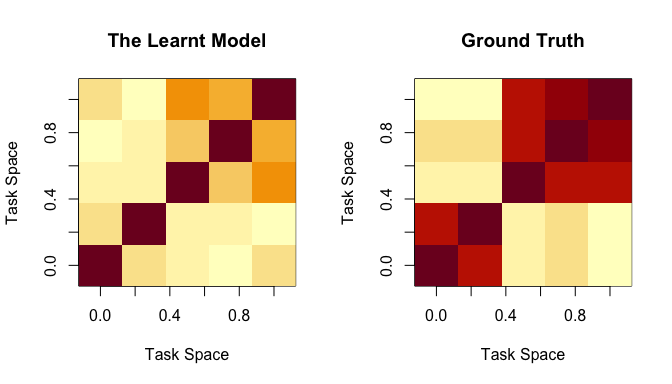# An Tutorial for Regularized Multi-task Learning using the package RMTL

Central Institute of Mental Health, Heidelberg University, Germany
library(RMTL)

## Abstract

This package provides an efficient implementation of regularized multi-task learning (MTL) comprising 10 algorithms applicable for regression, classification, joint feature selection, task clustering, low-rank learning, sparse learning and network incorporation (Cao, Zhou, and Schwarz 2018). All algorithms are implemented using the accelerated proximal algorithm and feature a complexity of O(1/k^2). In this tutorial, we show the theory and modeling of MTL with examples using the RMTL.

## Introduction

This package provides 10 multi-task learning algorithms (5 classification and 5 regression), which incorporate five regularization strategies for knowledge transferring among tasks. All algorithms share the same framework:

$\min\limits_{W, C} \sum_{i=1}^{t}{L(W_i, C_i |X_i, Y_i)} + \lambda_1\Omega(W) + \lambda_2{||W||}_F^2$ where $$L(\circ)$$ is the loss function (logistic loss for classification or least square loss for linear regression). $$\Omega(\circ)$$ is the cross-task regularization for knowledge transfer, and $$||W||_F^2$$ is used for improving the generalization. $$X=\{X_i= n_i \times p | i \in \{1,...,t\}\}$$ and $$Y=\{Y_i=n_i \times 1 | i \in \{1,...,t\}\}$$ are predictors matrices and responses of $$t$$ tasks respectively, while each task $$i$$ contains $$n_i$$ subjects and $$p$$ predictors. $$W=p \times t$$ is the coefficient matrix, where $$W_i$$ is the $$i$$th column of $$W$$ refers to the coefficient vector of task $$i$$. $$\Omega(W)$$ jointly modulates multi-task models($$\{W_1, W_2, ..., W_t\}$$) according to the specific prior structure. In this package, 5 common cross-task regularization methods are implemented to incorporate different priors, i.e. sparse structure, joint predictor selection, low-rank structure, network-based relatedness across tasks and task clustering. The mathematical formulation of each prior structure is demonstrated in the first row of Table 1. For all algorithms, we implemented an solver based on the accelerated gradient descent method, which takes advantage of information from the previous two iterations to calculate the current gradient and then achieves an improved convergent rate (Beck and Teboulle 2009). To solve the non-smooth and convex regularizer, the proximal operator is applied. Moreover, backward line search is used to determine the appropriate step-size in each iteration. Overall, the solver achieves a complexity of ($$\frac{1}{k^2}$$) and is optimal among first-order gradient descent methods.

All algorithms are summarized in Table 1. Each column corresponds to a MTL algorithm with an specific prior structure.To run an algorithm correctly, users need to tell RMTL the regularization type and problem type (regression or classification), which are summarized in the second and third row of Table 1. $$\lambda_1$$ aims to control the effect of cross-task regularization and could be estimated by the cross-validation (CV) procedure, and $$\lambda_2$$ is set by users in advance. Their valid ranges are summarized in the table. Please note, for $$\lambda_1=0$$, the effect of cross-task regularization was canceled. For the algorithm regularized by network and clustering prior (Graph and CMTL), extra information need to provide by users, i.e. $$G$$ encodes the network information and $$k$$ assumes the complexity of clustering structure. To train a multi-task model regularized by Lasso, Trace and L21, the sparsity is introduced by optimizing a non-smooth convex term. Therefore, the warm-start function is provided as part of the training procedure for these three algorithms. For this part, We will explain more details in the next section.

Table 1: Summary of Algorithms in RMTL
sparse structure joint feature learning low-rank structure network incorperation task clustering
$$\Omega(W)$$ $$||W||_1$$ $$||W||_{2,1}$$ $$||W||_*$$ $$||WG||_F^2$$ $$tr(W^TW)-tr(F^TW^TWF)$$
Regularization Type Lasso L21 Trace Graph CMTL
Problem Type R/C R/C R/C R/C R/C
$$\lambda_1$$ $$\lambda_1 > 0$$ $$\lambda_1 > 0$$ $$\lambda_1 > 0$$ $$\lambda_1 \geq 0$$ $$\lambda_1 > 0$$
$$\lambda_2$$ $$\lambda_2 \geq 0$$ $$\lambda_2 \geq 0$$ $$\lambda_2 \geq 0$$ $$\lambda_2 \geq 0$$ $$\lambda_2 > 0$$
Extra Input None None None G k
Warm Start Yes Yes Yes No No
Reference (Tibshirani 1996) (Liu, Ji, and Ye 2009) (Pong et al. 2010) (Widmer et al. 2014) (Jiayu Zhou, Chen, and Ye 2011)

## Cross-validation, Training and Prediction

For all algorithms in RMTL package, $$\lambda_1$$ illustrates the strength of relatedness of tasks and high value of $$\lambda_1$$ would result in highly similar models. For example, for MTL with low-rank structure (Trace), a large enough $$\lambda_1$$ will compress the task space to 1-dimension, thus coefficient vectors of all tasks are proportional. In RMTL, an appropriate $$\lambda_1$$ could be estimated using CV based on training data. $$\lambda_2$$ is suggested tuning manually by users with the default value of 0 (except for MTL with CMTL). The purpose of $$\lambda_2$$ is to introduce the penalty of quadratic form of $$W$$ leading to several benefits, i.e. promoting the grouping effect of predictors for selecting correlated predictors (Zou and Hastie 2005), stabilizing the numerical results and improving the generalization performance.

### Cross-validation

To run the cross-validation and training procedure successfully, the problem type and regularization type have to be given as the arguments to the function cvMTL(X, Y, Regularization=Regulrization, type=problem, …) for cross-validation and function MTL(X, Y, Regularization=Regulrization, type=problem …) for training, while the valid value of problem can be only “Regression” or “Classification” and the value of Regularization has to be selected from Table 1.

In the following example, we show how to use the function cvMTL(X, Y, …) for cross validation and demonstrate the selected parameter as well as CV accuracy curve.

#create simulated data
library(RMTL)
datar <- Create_simulated_data(Regularization="L21", type="Regression")

#perform the cross validation
cvfitr <- cvMTL(datar$X, datar$Y, type="Regression", Regularization="L21", Lam1_seq=10^seq(1,-4, -1),  Lam2=0, opts=list(init=0,  tol=10^-6, maxIter=1500), nfolds=5, stratify=FALSE, parallel=FALSE)

# meta-information and results of CV
#sequence of lam1
cvfitr$Lam1_seq #>  1e+01 1e+00 1e-01 1e-02 1e-03 1e-04 #value of lam2 cvfitr$Lam2
#>  0

#the output lam1 value with minimum CV error
print (paste0("estimated lam1: ", cvfitr$Lam1.min)) #>  "estimated lam1: 0.1" #plot CV errors across lam1 sequence in the log space plot(cvfitr)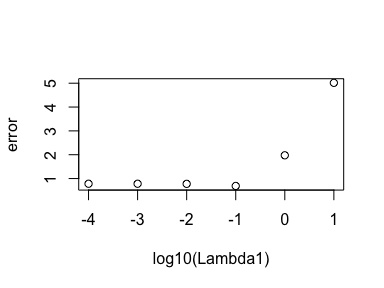Figure 1: CV errors across the sequence of $$\lambda_1$$ Stratified Cross-validation procedure is provided specific to the classification problem. The positive and negative subjects are uniformly distributed across folds such that the the performance of parameter selection is not biased by the data imbalance. To turn on the Stratified CV, users need to set cvfit(…,type=“Classification”, stratify=TRUE). Parallel Computing is allowed to speed up the CV procedure. To run the procedures of k folds simultaneously, the training data has to be replicated k times leading to the dramatically increased memory use. Therefore, users are suggested selecting less cores if the data size is large enough. To trigger on the parallel computing and select, i.e. 3 cores, one has to set cvfit(…,parallel=TRUE, ncores=3). In the following example, we show how to use Stratified CV and Parallel Computation in practice. We will compare the time consumption between the CV procedure with and without parallelization. Please note, in the Vignette, we are only allowed to use up to 2 cores for parallelization, thus the time comparison is less significant, users are suggested to use k cores in k-folds CV in practice. datac <- Create_simulated_data(Regularization="L21", type="Classification", n=100) # CV without parallel computing start_time <- Sys.time() cvfitc<-cvMTL(datac$X, datac$Y, type="Classification", Regularization="L21", stratify=TRUE, parallel=FALSE) Sys.time()-start_time #> Time difference of 0.517123 secs # CV with parallel computing start_time <- Sys.time() cvfitc<-cvMTL(datac$X, datac$Y, type="Classification", Regularization="L21", stratify=TRUE, parallel=TRUE, ncores=2) Sys.time()-start_time #> Time difference of 0.5581112 secs ### Training The result of CV (cvfit$Lam1.min) is sent to function MTL(X, Y, …) for training. After this, the coefficient matrices of all tasks {W, C} are obtained and ready for predicting new individuals. In the following example, we demonstrate the procedure of model training, the learnt parameters and the historical values of objective function.


#train a MTL model
model<-MTL(datar$X, datar$Y, type="Regression", Regularization="L21",
Lam1=cvfitr$Lam1.min, Lam2=0, opts=list(init=0, tol=10^-6, maxIter=1500), Lam1_seq=cvfitr$Lam1_seq)

#demo model
model
#>
#>              [,1]         [,2]         [,3]         [,4]          [,5]
#> [1,]  0.013627318 -0.004889863  0.001555228 -0.009912125 -0.0104298650
#> [2,] -0.038097508 -0.007636323 -0.001424447 -0.136329838 -0.0093494997
#> [3,] -0.105641820  0.022982948 -0.094069001  0.032763135  0.0057572766
#> [4,]  0.033725596 -0.084508343 -0.040979830  0.069090995  0.0240164403
#> [5,] -0.004595257 -0.005870497 -0.009110698 -0.028671679 -0.0002629989
#> [6,]  0.069280736  0.088372444 -0.050309117 -0.037685263 -0.0903658014
#> Call:
#> MTL(X = datar$X, Y = datar$Y, type = "Regression", Regularization = "L21",
#>     Lam1 = cvfitr$Lam1.min, Lam1_seq = cvfitr$Lam1_seq, Lam2 = 0,
#>     opts = list(init = 0, tol = 10^-6, maxIter = 1500))
#> type:
#>  "Regression"
#> Formulation:
#>  "SUM_i Loss_i(W) + Lam1*||W||_{2,1} + Lam2*||W||{_2}{^2}"

# learnt models {W, C}
head(model$W) #> [,1] [,2] [,3] [,4] [,5] #> [1,] 0.013627318 -0.004889863 0.001555228 -0.009912125 -0.0104298650 #> [2,] -0.038097508 -0.007636323 -0.001424447 -0.136329838 -0.0093494997 #> [3,] -0.105641820 0.022982948 -0.094069001 0.032763135 0.0057572766 #> [4,] 0.033725596 -0.084508343 -0.040979830 0.069090995 0.0240164403 #> [5,] -0.004595257 -0.005870497 -0.009110698 -0.028671679 -0.0002629989 #> [6,] 0.069280736 0.088372444 -0.050309117 -0.037685263 -0.0903658014 head(model$C)
#>  -0.30989788  0.31574605 -0.22983163 -0.04739195 -0.29785363

# Historical objective values
str(model$Obj) #> num [1:75] 1.99 1.63 1.44 1.35 1.3 ... # other meta infomration model$Regularization
#>  "L21"
model$type #>  "Regression" model$dim
#>      [,1] [,2] [,3] [,4] [,5]
#> [1,]   20   20   20   20   20
#> [2,]   50   50   50   50   50
str(model$opts) #> List of 5 #>$ init   : num 1
#>  $tol : num 1e-06 #>$ maxIter: num 1500
#>  $W0 : num [1:50, 1:5] 0.0136 -0.0381 -0.1056 0.0337 -0.0046 ... #>$ C0     : num [1:5] -0.3099 0.3157 -0.2298 -0.0474 -0.2979

#plot the historical objective values in the optimization
plotObj(model)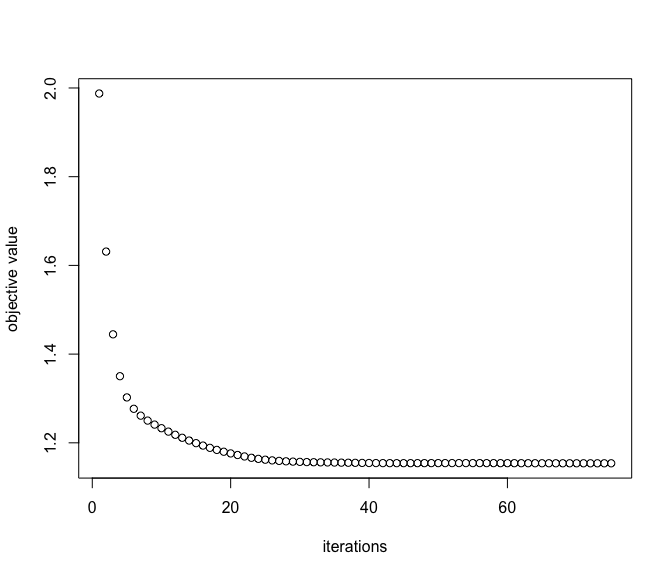Figure 2: The convergence of objective values across iterations

### Prediction and Error Calculation

To predict the new individuals, two functions are relevant, predict() and calcError(). predict() is used to calculate the outcome given the data of new individuals. Especially, for classification, the outcome is represented as the probability of the individual being assigned to the positive label ($$P(Y==1)$$). calcError() is used to test if the model works well by calculating the prediction error rate. According to the problem type, specific metric is used, for example, the miss-classification rate ($$\frac{1}{n} \sum_i^n 1_{\hat{y}_i=y_i}$$) is used for classification problem and the mean square error (MSE) ($$\sum_i^n (\hat{y}_i-y_i)^2$$) is used for the regression problem. In the following examples, we will show you how to calculate the training and test error, and then make predictions for both classification and regression problem. To achieve it, we have to create the simulated data and train the models in advance just like we did before.

# create simulated data for regression and classification problem
datar <- Create_simulated_data(Regularization="L21", type="Regression")
datac <- Create_simulated_data(Regularization="L21", type="Classification")

# perform CV
cvfitr<-cvMTL(datar$X, datar$Y, type="Regression", Regularization="L21")
cvfitc<-cvMTL(datac$X, datac$Y, type="Classification", Regularization="L21")

# train
modelr<-MTL(datar$X, datar$Y, type="Regression", Regularization="L21",
Lam1=cvfitr$Lam1.min, Lam1_seq=cvfitr$Lam1_seq)
modelc<-MTL(datac$X, datac$Y, type="Classification", Regularization="L21",
Lam1=cvfitc$Lam1.min, Lam1_seq=cvfitc$Lam1_seq)

# test
# for regression problem
calcError(modelr, newX=datar$X, newY=datar$Y) # training error
#>  0.0001667676
calcError(modelr, newX=datar$tX, newY=datar$tY) # test error
#>  0.7359266
# for calssification problem
calcError(modelc, newX=datac$X, newY=datac$Y) # training error
#>  0
calcError(modelc, newX=datac$tX, newY=datac$tY) # test error
#>  0.23

# predict
str(predict(modelr, datar$tX)) # for regression #> List of 5 #>$ : num [1:20, 1] 1.511 -1.304 -2.692 -2.58 0.255 ...
#>  $: num [1:20, 1] 2.04 1.67 5.14 2.93 3.35 ... #>$ : num [1:20, 1] 0.491 -0.874 -0.218 1.275 -3.16 ...
#>  $: num [1:20, 1] 0.125 -2.25 1.392 2.551 5.587 ... #>$ : num [1:20, 1] -2.383 -1.537 0.954 -0.758 1.39 ...
str(predict(modelc, datac$tX)) # for classification #> List of 5 #>$ : num [1:20, 1] 0.72 0.217 0.664 0.754 0.674 ...
#>  $: num [1:20, 1] 0.99 0.961 0.83 0.986 0.743 ... #>$ : num [1:20, 1] 0.5637 0.9918 0.7815 0.0874 0.8294 ...
#>  $: num [1:20, 1] 0.0582 0.067 0.2284 0.0589 0.6164 ... #>$ : num [1:20, 1] 0.403 0.159 0.937 0.326 0.358 ...

### Control of Optimization

Options are provided to control the optimization procedure using the argument opts=list(init=0, tol=10^-6, maxIter=1500). opts$init specifies the starting point of the gradient descent algorithm, opts$tol controls tolerance of the acceptable precision of solution to terminate the algorithm, and opts$maxIter is the maximum number of iterations. These options can be modified by users for adapting to the scale of problem and computing resource. For opts$init, two options are provided. opts$init=0 refers to $$0$$ matrix. And opts$init=1 refers to the user-specific starting point. If specified, the algorithm will attempt to access opts$W0 and opts$C0 as the starting point, which has to be given by users in advance. Otherwise, errors are reported. Particularly, the setting opts$init=1 is key to warm-start technique for sparse model training. In the following example, we aim to calculate a highly precise solution by restricting the tolerance and increasing the number of iterations. In the left figure, the precision of the solution is 0.02 and the algorithm stops after <20 iterations. In the right figure, the precision is set to 10^-8 and the algorithm takes more iterations to run. par(mfrow=c(1,2)) model<-MTL(datar$X, datar$Y, type="Regression", Regularization="L21", Lam1=cvfitr$Lam1.min, Lam2=0, opts=list(init=0,  tol=10^-2,
maxIter=10))
plotObj(model)
model<-MTL(datar$X, datar$Y, type="Regression", Regularization="L21",
Lam1=cvfitr$Lam1.min, Lam2=0, opts=list(init=0, tol=10^-8, maxIter=100)) plotObj(model)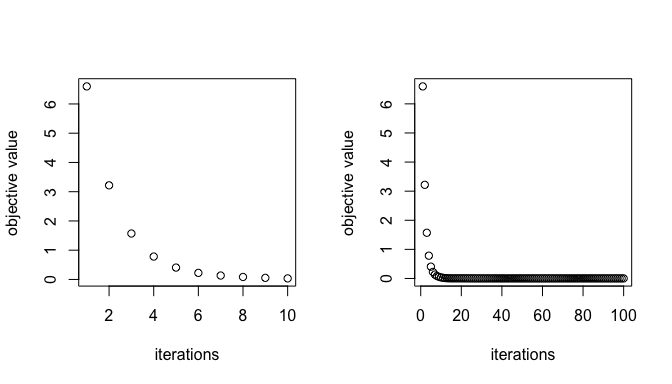Figure 3: Calculate rough (Left) and precise (Right) by controling the optimization warm-start and cold-start are both provided to train a sparse model. The reason for warm-start is due to the non_strict convexity of the objective function, more than one (usually unlimited) number of optimal solutions exist, which made the model difficult to interpret. In the warm-start, $$\lambda_1$$ sequence ($$\{...,\lambda_1^{(i)},\lambda_1^{(i-1)},...\}$$) is feed to the training procedure in the decreasing order. Meanwhile, the solution of $$\lambda_1^{(i)}$$, $$\{\overset{*}{W}^{(i)}, \overset{*}{C}^{(i)}\}$$, is set as the initial point of the $$\lambda_1^{(i-1)}$$, which leads to a unique solution path. In the example, we investigate the algorithm of multi-task feature selection and draw a regularization tree to show the model interpretation. In Figure 4, each line represents the change of significance of one predictor across the $$\lambda_1$$ sequence. By relaxing $$\lambda_1$$, more predictors are allowed to contribute to the prediction. Please note, significance per predictor is calculated as the euclidean norm of parameters across tasks. Lam1_seq=10^seq(1,-4, -0.1) opts=list(init=0, tol=10^-6,maxIter=100) mat=vector() for (i in Lam1_seq){ m=MTL(datar$X, datar$Y, type="Regression", Regularization="L21", Lam1=i,opts=opts) opts$W0=m$W opts$C0=m$C opts$init=1
mat=rbind(mat, sqrt(rowSums(m$W^2))) } matplot(mat, type="l", xlab="Lambda1", ylab="Significance of Each Predictor")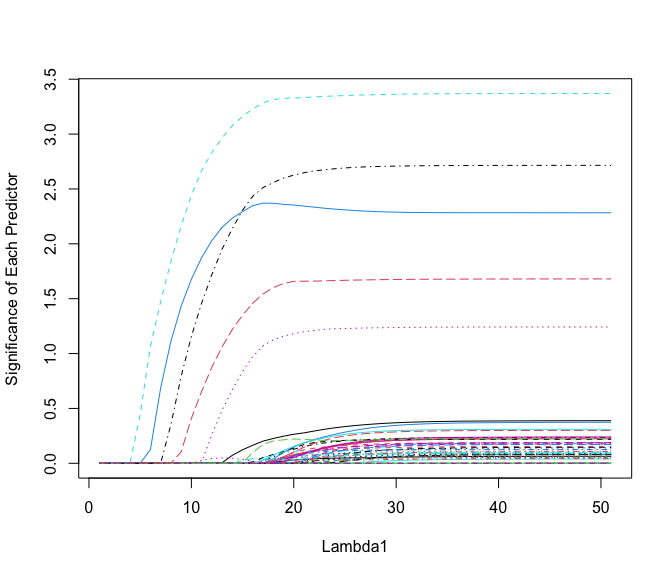Figure 4: The solution path using warm-start The warm-start is triggered by sending two arguments ($$\lambda_1$$, $$\lambda_1$$ sequence) to the function MTL(…, Lam1=Lam1_value, Lam1_seq=Lam1_sequence), and they can be calculated by the CV procedure or set manually. However, if only Lam1=Lam1_value is given, the sparsity is introduced using cold-start (the initial point is set only via opts$init). See examples below, different setting leads to different solution.

#warm-start
model1<-MTL(datar$X, datar$Y, type="Regression", Regularization="L21", Lam1=0.01, Lam1_seq=10^seq(0,-4, -0.1))
str(model1$W) #> num [1:50, 1:5] 0.027774 -0.120535 0.000559 0.143741 -0.027749 ... #cold-start model2<-MTL(datar$X, datar$Y, type="Regression", Regularization="L21", Lam1=0.01) str(model2$W)
#>  num [1:50, 1:5] -0.00801 -0.29367 0.15996 0.09473 -0.05239 ...

### Data Simulation Methods

Create_simulated_data(…) is used to generate examples for testing every MTL algorithm. To create datasets of variant number of tasks, subjects and predictors, the function accepts several arguments: $$t$$, $$p$$ and $$n$$ illustrating the number of tasks, predictors and subjects. Here, for convenience, we only allow same number of subjects for all tasks. To test the specific functionality of each MTL algorithm, the simulation datasets are generated with the specific prior structure. For details of this part, users are suggested to check out the supplementary of the original paper (Cao, Zhou, and Schwarz 2018). The problem type and regularization type need to be specific as the arguments to create an algorithm-specific dataset. Finally, 5 objects are outputted: $$(X, Y, tX, tY, W)$$, where $$(X, Y)$$ are used for training, $$(tX, tY)$$ are used for testing, and $$W$$ is the ground truth model. In addition, for multi-task algorithms regularized by Graph and CMTL, extra information is also generated and outputted i.e. matrix $$G$$ and $$k$$.

In the following example, we demonstrate how to create datasets to test the MTL regression method with low-rank structure (Trace), including 100 tasks, as well as 10 subjects, 20 predictors for each task.

data <- Create_simulated_data(Regularization="Trace", type="Regression", p=20, n=10, t=100)
names(data)
#>  "X"  "Y"  "tX" "tY" "W"
length(data$X) #>  100 # number of subjects and predictors dim(data$X[])
#>  10 20

$\min\limits_{W, C} \sum_{i=1}^{t}{L(W_i, C_i|X_i, Y_i)} + \lambda_1\Omega(W) + \lambda_2{||W||}_F^2$

Recall the shared formulation of all algorithms in RMTL, loss function $$L(\circ)$$ could be logistic loss for classification problem $L(W_i, C_i)= \frac{1}{n_i} \sum_{j=1}^{n_i} log(1+e^{-Y_{i,j}(X_{i,j}W_i^T+C_i)})$ or least square loss for regression problem. $L(W_i, C_i)= \frac{1}{n_i} \sum_{j=1}^{n_i} ||Y_{i,j}-X_{i,j}W_i^T-C_i||^2_2$ where $$i$$ indexes tasks and and $$j$$ indexes subjects in each task, therefore $$Y_{i,j}$$ and $$X_{i,j}=1 \times p$$ refer to the outcome and predictors of subject $$j$$ in task $$i$$, while $$n_i$$ refer to the number of subjects in task $$i$$.

In the following sections, we will show you how to train a multi-task model with specific cross-task regularization and how to interpret it. Please note, in the Vignettes of R, we are only allowed to demonstrate algorithms on small datasets leading to the less significant results (especially limiting the performance of cross-validation procedure). For the complete analysis, users are directed to the original paper (Cao, Zhou, and Schwarz 2018).

### MTL with sparse structure

$\min\limits_{W, C} \sum_{i=1}^{t}{L(W_i, C_i|X_i, Y_i)} + \lambda_1||W||_1 + \lambda_2{||W||}_F^2$ This formulation extends the lasso (Tibshirani 1996) to the multi-task scenario such that all models are penalized according to the same $$L_1$$ strength, and all unimportant coefficients are shrunken to 0, to achieve the global optimum. $$||\circ||_1$$ is the $$L_1$$ norm.

In the example, we train and test this algorithm and demonstrate the model in the regression problem.

#create data
data <- Create_simulated_data(Regularization="Lasso", type="Regression")
#CV
cvfit<-cvMTL(data$X, data$Y, type="Regression", Regularization="Lasso")
cvfit$Lam1.min #>  0.1 #Train m=MTL(data$X, data$Y, type="Regression", Regularization="Lasso", Lam1=cvfit$Lam1.min, Lam1_seq=cvfit$Lam1_seq) #Test paste0("test error: ", calcError(m, data$tX, data$tY)) #>  "test error: 18.5132932581855" #Show models par(mfrow=c(1,2)) image(t(m$W), xlab="Task Space", ylab="Predictor Space")
title("The Learnt Model")
image(t(data$W), xlab="Task Space", ylab="Predictor Space") title("The Ground Truth")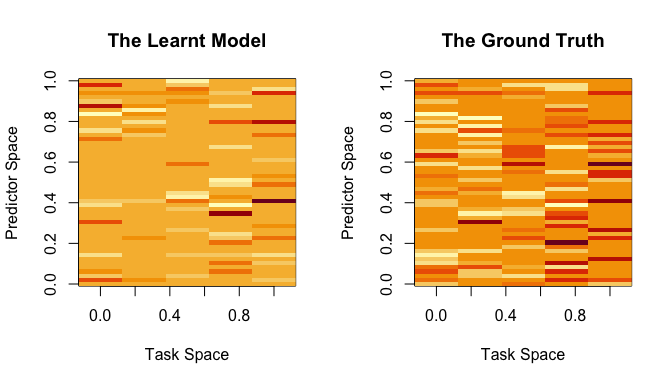Figure 5: Comparision of $$\hat{W}$$ and $$W$$ In Figure 5, the learnt model is more sparse than the ground truth due to the fact that $$L_1$$ penalty only selects one from correlated predictors such that most correlated predictors are penalized to 0 (Zou and Hastie 2005). In this case, users are suggested to use $$\lambda_2$$ to turn on the multi-task learning algorithm with elastic net. More analysis could be found in the original paper (Cao, Zhou, and Schwarz 2018). ### MTL with joint predictor selection $\min\limits_{W, C} \sum_{i=1}^{t}{L(W_i, C_i|X_i, Y_i)} + \lambda_1||W||_{2,1} + \lambda_2{||W||}_F^2$ This formulation constraints all models to select or reject the same set of features simultaneously. Therefore, the solution only contains predictors which are consistently important to all tasks. $$||W||_{2,1}=\sum_k||W_{k,:}||_2$$ aims to create the group sparse structure in the feature space. In the multi-task learning scenario, the same feature of all tasks form a group (each row of $$W$$), such that features in the same group are equally penalized, while results in the group-wise sparsity. This approach has been used in the transcriptomic markers mining across multiple platforms, where the common set of biomarkers are shared (Xu, Xue, and Yang 2011). The approach observed the improved prediction performance compared to the single-task learning methods. #create datasets data <- Create_simulated_data(Regularization="L21", type="Regression") #CV cvfit<-cvMTL(data$X, data$Y, type="Regression", Regularization="L21") #Train m=MTL(data$X, data$Y, type="Regression", Regularization="L21", Lam1=cvfit$Lam1.min, Lam1_seq=cvfit$Lam1_seq) #Test paste0("test error: ", calcError(m, data$tX, data$tY)) #>  "test error: 0.90725817488304" #Show models par(mfrow=c(1,2)) image(t(m$W), xlab="Task Space", ylab="Predictor Space")
title("The Learnt Model")
image(t(data$W), xlab="Task Space", ylab="Predictor Space") title("The Ground Truth")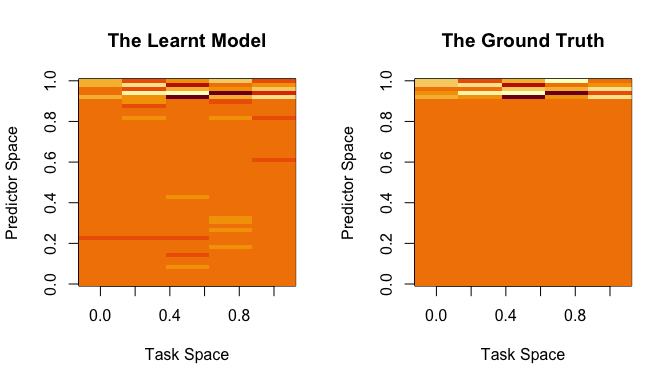Figure 6: Comparision of $$\hat{W}$$ and $$W$$ In the Figure 6, the ground truth model consists of top 5 active predictors while all others are 0. By assuming all tasks sharing the same set of predictors, MTL with L21 successfully locates most true predictors. The complete analysis could be found here (Cao, Zhou, and Schwarz 2018). ### MTL with low-rank structure $\min\limits_{W, C} \sum_{i=1}^{t}{L(W_i, C_i|X_i, Y_i)} + \lambda_1||W||_* + \lambda_2{||W||}_F^2$ This formulation constraints all models to a low-rank subspace. With increasing penalty($$\lambda_1$$), the correlation between models increases. $$||W||_*$$ is the trace norm of $$W$$. This method has been applied to improve the drug sensitivity prediction by combining multi-omic data, which successfully captures the information of drug mechanism of action using the low-rank structure (Yuan et al. 2016). #create data data <- Create_simulated_data(Regularization="Trace", type="Classification") #CV cvfit<-cvMTL(data$X, data$Y, type="Classification", Regularization="Trace") #Train m=MTL(data$X, data$Y, type="Classification", Regularization="Trace", Lam1=cvfit$Lam1.min, Lam1_seq=cvfit$Lam1_seq) #Test paste0("test error: ", calcError(m, data$tX, data$tY)) #>  "test error: 0.3" #Show task relatedness par(mfrow=c(1,2)) image(cor(m$W), xlab="Task Space", ylab="Task Space")
title("The Learnt Model")
image(cor(data$W), xlab="Task Space", ylab="Task Space") title("The Ground Truth")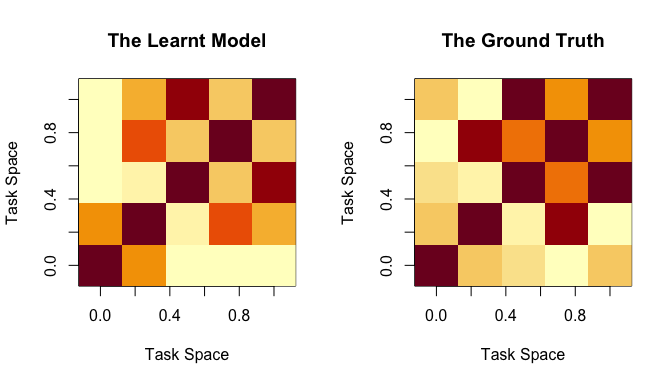Figure 7: Comparision of the learnt task relatedness and ground truth To interpret this model, we show the correlation coefficient of pairwise models as shown in the Figure 7. Through this comparison, users could check if the task relatedness is well captured. ### MTL with network structure $\min\limits_{W, C} \sum_{i=1}^{t}{L(W_i, C_i|X_i, Y_i)} + \lambda_1||WG||_F^2 + \lambda_2{||W||}_F^2$ This formulation constraints the models’ relatedness according to the pre-defined graph . If the penalty is heavy enough, the difference of connected tasks is 0. Network matrix $$G$$ has to be modeled by users. Intuitively, $$||WG||_F^2$$ equals to an accumulation of differences between related tasks, i.e. $$||WG||_F^2=\sum ||W_{\tau}-W_{\phi}||_F^2$$, where $$\tau$$ and $$\phi$$ are closely connected tasks over an network. In general, given an undirected graph representing the network, $$||WG||_F^2=tr(WLW^T)$$, where $$L$$ is the graph Laplacian. Therefore, penalizing such term improves the task relatedness. However, it is nontrivial to design $$G$$. Here, we give three common examples to demonstrate the construction of $$G$$. 1), Assume your tasks are subject to orders i.e. temporal or spatial order. Such order forces the order-oriented smoothness across tasks such that the adjacent models(tasks) are related, then the penalty can be designed as: $||WG||_F^2 = \sum_{i=1}^{t-1}||W_i-W_{i+1}||_F^2$ where $$G=(t+1)\times t$$ $G_{i,j} = \begin{cases} 1 & i=j \\ -1 & i=j+1 \\ 0 & others \end{cases}$ 2), The so-called mean-regularized multi-task learning (Evgeniou and Pontil 2004). In this formulation, each model is forced to approximate the mean of all models. $||WG||_F^2 = \sum_{i=1}^{t}||W_i - \frac{1}{t} \sum_{j=1}^t W_j||_F^2$ where $$G = t \times t$$ $G_{i,j} = \begin{cases} \frac{t-1}{t} & i=j \\ \frac{1}{t} & others \end{cases}$ 3), Assume your tasks are related according to a given graph $$g=(N,E)$$ where $$E$$ is the edge set, and $$N$$ is the node set. Then the penalty is $||WG||_F^2 = \sum_{\alpha \in N, \beta \in N, (\alpha, \beta) \in E}^{||E||} ||W_{:, \alpha} - W_{:,\beta}||_F^2$ where $$\alpha$$ and $$\beta$$ are connected tasks in the network. $$G=t \times ||E||$$. $G_{i, j} = \begin{cases} 1 & i=\alpha^{(j)} \\ -1 & j=\beta^{(j)} \\ 0 & others \end{cases}$ where $$(\alpha^{(j)}, \beta^{(j)})$$ is the $$j$$th term of set $$E$$. In the bio-medical applications, all three constructions are beneficial. The first construction was used for predicting the disease progression of Alzheimer by holding the assumption of temporal smoothness, which successfully identified the progression-related biomarkers (J. Zhou et al. 2013). The second the construction is applied to explore the transcriptomic biomarkers and risk prediction for schizophrenia by integrating heterogeneous transcriptomic datasets, which demonstrates the strong interpretability of MTL method in multi-modal data analysis (Cao, Meyer-Lindenberg, and Schwarz 2018). The third construction has been used in the recognition of splice sites in genome leading to an improved the prediction performance by considering the task-task relatedness (Widmer et al. 2010). In the following example, we create 5 tasks forming 2 groups(first group: first two tasks; second group: last three tasks). The tasks are highly correlated within the group and show no group-wise correlation. We will show you how to embed the known task-task relatedness in MTL. #create datasets data <- Create_simulated_data(Regularization="Graph", type="Classification") #CV cvfit<-cvMTL(data$X, data$Y, type="Classification", Regularization="Graph", G=data$G)
#Train
m=MTL(data$X, data$Y, type="Classification", Regularization="Graph", Lam1=cvfit$Lam1.min, Lam1_seq=cvfit$Lam1_seq, G=data$G) #Test print(paste0("the test error is: ", calcError(m, newX=data$tX, newY=data$tY))) #>  "the test error is: 0.38" #Show task relatedness par(mfrow=c(1,2)) image(cor(m$W), xlab="Task Space", ylab="Task Space")
title("The Learnt Model")
image(cor(data$W), xlab="Task Space", ylab="Task Space") title("The Ground Truth")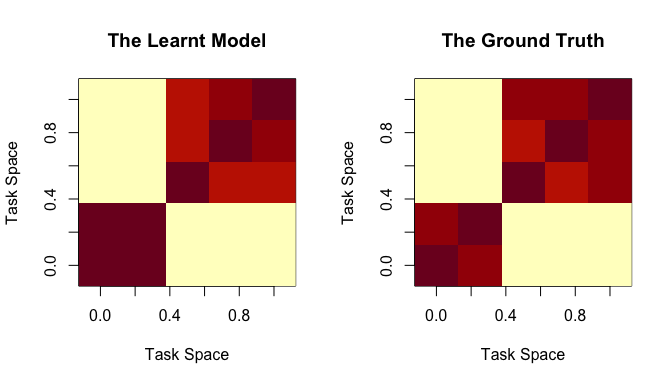Figure 8: Compare the Learnt Task Relatedness with the Ground Truth ### MTL with clustering structure $\min\limits_{W, C, F: F^TF=I_k} \sum_{i=1}^{t}{L(W_i, C_i|X_i, Y_i)} + \lambda_1 (tr(W^TW)-tr(F^TW^TWF)) + \lambda_2{||W||}_F^2$ The formulation combines the data fitting term ($$L(\circ)$$) and the loss function of k-means clustering ($$(tr(W^TW)-tr(F^TW^TWF)$$), and therefore is able to detect a clustered structure among tasks. $$\lambda_1$$ is used to balance the clustering and data fitting effects. However the above formulation is non-convex form leading to the challenge for reaching the global optimum, therefore we implements the relaxed convex form of the formulation (Jiayu Zhou, Chen, and Ye 2011). $\min\limits_{W, C, F: F^TF=I_k} \sum_{i=1}^{t}{L(W_i, C_i|X_i, Y_i)} + \lambda_1 \eta (1+\eta)||W(\eta I+M)^{-1}W^T||_*$ $S.T. \enspace tr(M)=k, S \preceq I, M \in S^t_+, \eta=\frac{\lambda_2}{\lambda_1}$ where $$S_+^t=t \times t$$ denotes the symmetric positive semi-definite matrices, $$S \preceq I$$ restricts $$I-M$$ to be positive semi-definite. $$M=t \times t$$ reflects the learnt clustered structure, k refers to the complexity of the structure. In the relaxed form, $$\lambda_2=0$$ leads to the $$\Omega(W)=0$$ which canceled the effect of cross-task regularization, therefore $$\lambda_2>0$$ is suggested. In the following example, 5 tasks forms two clusters, and we will show you how to train a MTL model while capturing the clustering structure. To demonstrate the result, the task-task correlation matrix is shown in the Figure 9 #Create datasets data <- Create_simulated_data(Regularization="CMTL", type="Regression") cvfit<-cvMTL(data$X, data$Y, type="Regression", Regularization="CMTL", k=data$k)
m=MTL(data$X, data$Y, type="Regression", Regularization="CMTL", Lam1=cvfit$Lam1.min, Lam1_seq=cvfit$Lam1_seq, k=data$k) #Test paste0("the test error is: ", calcError(m, newX=data$tX, newY=data$tY)) #>  "the test error is: 41.7059285869177" #Show task relatedness par(mfrow=c(1,2)) image(cor(m$W), xlab="Task Space", ylab="Task Space")
title("Ground Truth")